### IMO Shortlist 2012 problem G4

Kvaliteta:
Avg: 4,0
Težina:
Avg: 7,0
Let$ABC$ be a triangle with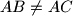$AB \neq AC$ and circumcenter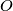$O$. The bisector of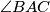$\angle BAC$ intersects$BC$ at$D$. Let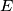$E$ be the reflection of$D$ with respect to the midpoint of$BC$. The lines through$D$ and$E$ perpendicular to$BC$ intersect the lines$AO$ and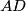$AD$ at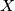$X$ and$Y$ respectively. Prove that the quadrilateral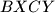$BXCY$ is cyclic.
Izvor: Međunarodna matematička olimpijada, shortlist 2012